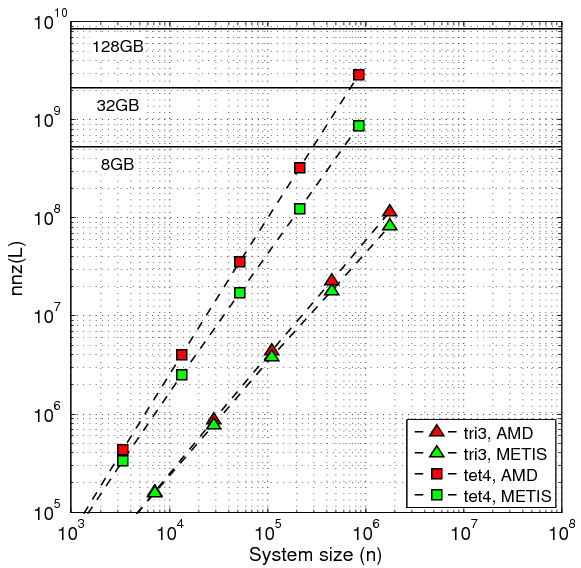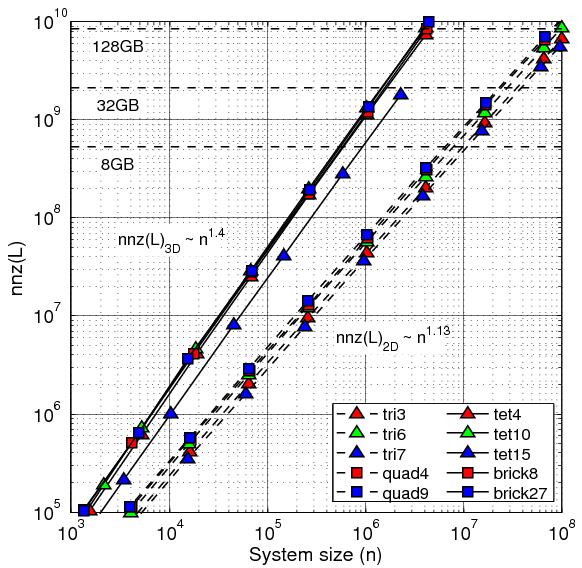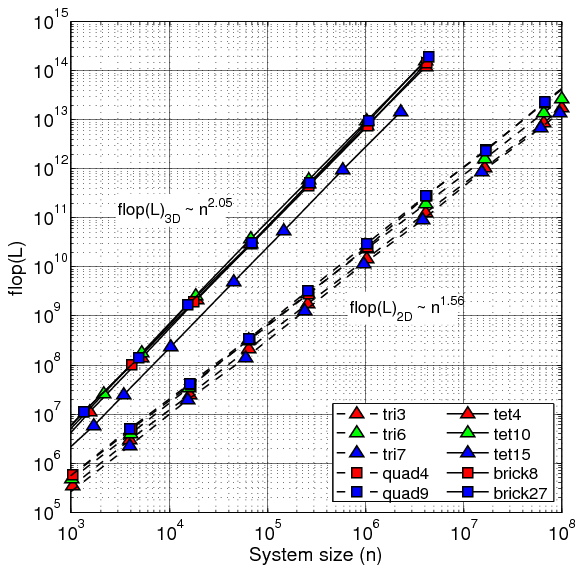# Reordering

Direct solvers of linear systems of equations factorize the system matrix A into a product of lower- and upper-triangular matrices, e.g. A=L*U in case of LU factorization, or A=L*LT in case of Cholesky factorization. Although for the problems considered here the system matrix A is sparse (nnz(A) << n2), usually the number of non-zero entries in the factors is significantly larger than in the system matrix. New non-zero entries are added in the factors compared to the system matrix A and in the worst case scenario the factors can be dense triangular matrices.

The number of the additional non-zero entries introduced during the factorization, often referred to as the fill-in, depends on the structure of A. Renumbering of the unknowns prior to the factorization alters the non-zero structure (the sparsity pattern) of A and therefore affects the fill-in. The aim of the reordering step is to find a permutation of the unknowns that minimizes the fill-in. To summarize, the fill-reducing reordering is crucial since it decreases both memory and computational requirements:

• The fill-in determines the amount of memory required to store the factors. If the memory requirements are larger than the available RAM the factorization will either fail, or will be significantly slower due to swapping.
• The amount fill-in affects the flop count, and consequently the computational time required to perform the factorization.
• The number of non-zero entries in the factors affects the flop count and the computational time required to perform the backward and forward substitution.

Finding the optimal reordering that would result in a minimum fill-in is an NP-hard problem. Essentially, this means that for a general sparse matrix there is no efficient algorithm to find the best permutation and effectively all of the permutations need to be checked. Clearly, this is not practical even for systems with hundred unknowns. Various heuristic reordering techniques have been developed that aim at decreasing the fill-in during sparse factorization well enough and in a reasonable time. In this section we present a comparison of the AMD and METIS algorithms. AMD (Approximate Minimum Degree) is an built-in MATLAB routine, while METIS is accessible in MATLAB through the SuiteSparse package. These techniques are very effective for matrices resulting from discretization of PDEs using local methods.

#### Reordering, Fill-in, and Flop Count during factorization

Figure 1 presents a comparison of the effectiveness of AMD and METIS reorderings. The number of non-zero entries in the factor nnz(L) is shown as a function of the system size for 2D and 3D domains discretized with 3-node triangles and 4-node tetrahedrons, respectively. In the log-log space nnz(L) can be fitted by a linear function of the number of mesh nodes n. This means that nnz(L) is a power-law function of n

nnz(L) = nα

The larger the exponent α, the faster the number of non-zero entries in the factor grows with the system size. In practice this means that lower exponents allow larger systems to be solved on a computer with a given memory configuration configuration. In the shown comparison the exponent is larger for AMD reordering in both 2D and 3D: 1.19 and 1.59, respectively, compared to 1.13 and 1.42 obtained using METIS.Figure 1. Number of non-zero entries in the factor for AMD and METIS reorderings. Matrices resulting from FEM meshes with 2D 3-node triangular elements and 3D 4-node tetrahedral elements. One degree of freedom per mesh node.

Figure 2 presents nnz(L) as a function of the system size n for two- and three-dimensional meshes and for various elements. Only METIS reordering is shown. Sparse matrices considered result from Finite Element discretization of the Poisson’s equation (1 degree of freedom per mesh node). The power-law exponent is differen in two and three dimensions, but similar for different element types.Figure 2. Number of non-zero entries in the factor depending on spatial dimension of the mesh, system size and element type. One degree of freedom per mesh node. METIS fill-reducing reordering. Memory requirements for the factors assuming MATLAB sparse storage marked for reference.

Figure 3 presents the number of floating point operations (flop count) required to perform the factorization of the global stiffness matrix after reordering. Also in this case the number of flops required is a power-law function of the system size. The table below summarizes the power-law exponents for matrices resulting from 2D and 3D problems averaged for different element types.Figure 3. Flop count of the Cholesky factorization depending on spatial dimension of the mesh, system size and element type. One degree of freedom per mesh node. METIS fill-reducing reordering.

 2D 3D nnz(L) flop count nnz(L) flop count AMD METIS 1.13 1.56 1.40 2.06 Theoretical bounds n log(n) 1.50 1.33 2.00

#### A system size of one million nodes

As seen in Figure 1, for systems with around 1 million nodes in 2D the difference in memory consumption is not very signifficant. The memory usage due to the factor is 1.7 GB and 1.2 GB for AMD and METIS reorderings, respectively. The difference shows much more in 3D: with AMD the factor uses 43 GB of RAM, while with METIS only 13 GB.

For a system size of one million degrees of freedom in 2D the number of non-zero entries in the factor resulting from the METIS reordering is approximately two times lower than that obtained using AMD. The difference is approximately five times for the 3D problems. Considering the flop count, for 2D and 3D problems METIS results in 2 times and 15 times less work, respectively.

#### CPU Time Required to Compute Reordering

The reordering step is itself a rather costly operation. The time required to find the permutation of the unknowns should be considered in view of the number of times the permutation vectors can be reused. Note that the permutation only needs to be computed when the mesh topology changes. Hence, whether a best possible reordering is crucial, or whether the reordering time also contributes significantly to the total solution time depends on the problem at hand.

Figure TODO shows the CPU time required to find the reordering using AMD and METIS. Reordering is computed using sparse matrices resulting from two-dimensional meshes of 7-node triangular elements, one degree of freedom per node. Note that the CPU time spent on the reordering scales non-linearly with the system size, with power-law exponents varying from 1.06 – 1.16 for both reordering schemes. AMD appears to be generally more efficient than METIS, with approximately 6 times shorter CPU times for all system sizes.

#### Summary of the Comparison of AMD and METIS Reordering Schemes

We have presented the results from three different studies, in which we compared the performance of the AMD and METIS reordering schemes. While it takes less CPU time to compute the reordering using AMD scheme, it results in a larger number of operations (nop) required to perform the Cholesky factorization, as well as higher fill-in (nnz) of the matrix. The benefit from using METIS, in terms of nop and nnz, is higher for larger system sizes, as a result of super-linear scaling, and is especially significant for the three-dimensional problems. If the reordering can be reused for a large number of steps, it is recommended to use the METIS scheme.

#### Application of the Reordering to the Global System of Linear Equations

In this subsection we discuss two different approaches to apply the computed permutation vectors to the global stiffness matrix, only the lower part of which is stored. First approach is presented in Code Fragment 1, and utilizes the external libraries cs_transpose and cs_symperm, provided by the Suite Sparse package. The second approach is presented in Code Fragment 2, and only utilizes the inbuilt MATLAB-routines. The computational time required to carry out each of these code fragments, as a function of system size, is presented in the figure below. We observe that the Code Fragment 2 is more efficient, with an increasing gain for larger system sizes.

Code Fragment 1:

``` A = cs_transpose(A); A = cs_symperm(A,perm); A = cs_transpose(A);```

Code Fragment 2:

``` A = A(perm,perm); A = tril(A) + triu(A,1)';```